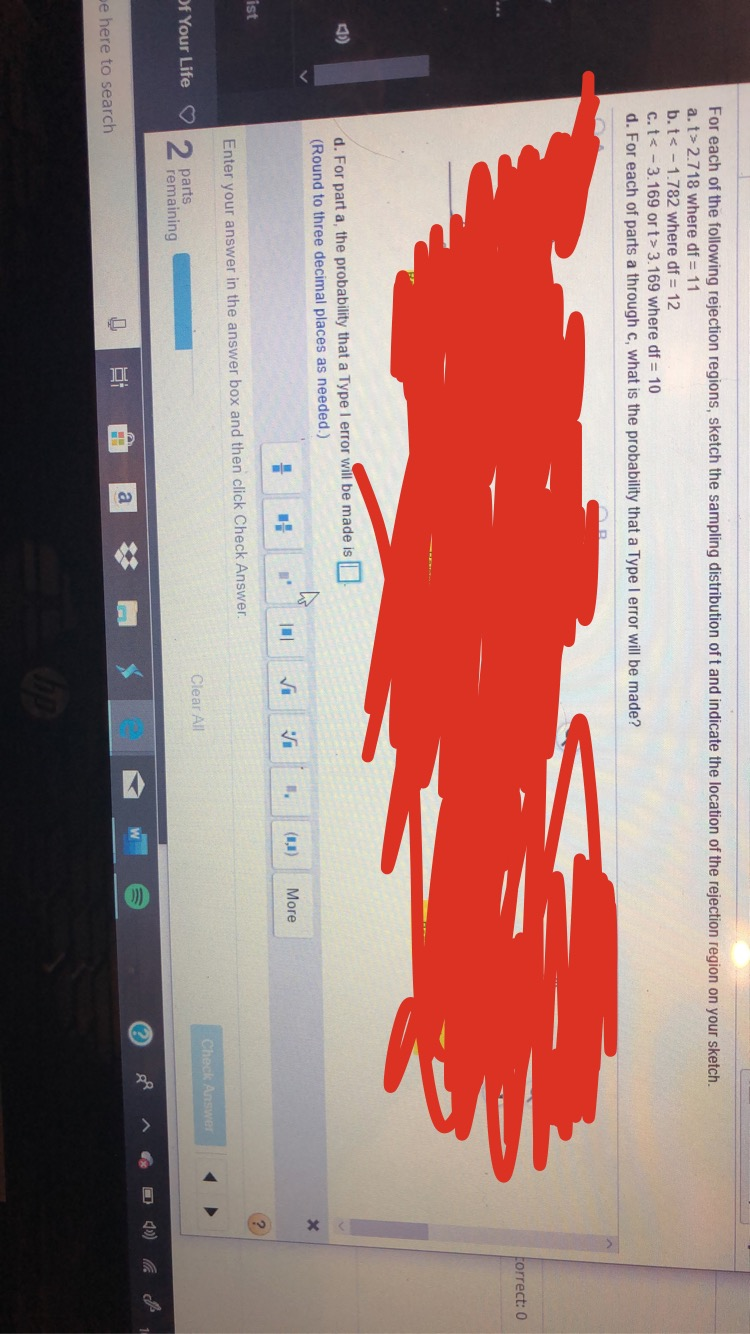# For each of the following rejection regions, sketch the sampling distribution of t and indicate the location of the rejection region on your sketch.a. t> 2.718 where df 11b.t< -1.782 where df 12c. t< -3.169 or t> 3.169 where df10d. For each of parts a through c, what is the probability that a Type I error will be made?torrect: 0d. For part a, the probability that a Type I error will be made is(Round to three decimal places as needed.))MoreistEnter your answer in the answer box and then click Check Answer2 partsremainingOf Your LifeClear AllCheck Answee here to search

Question
98 viewshelp_outlineImage TranscriptioncloseFor each of the following rejection regions, sketch the sampling distribution of t and indicate the location of the rejection region on your sketch. a. t> 2.718 where df 11 b.t< -1.782 where df 12 c. t< -3.169 or t> 3.169 where df 10 d. For each of parts a through c, what is the probability that a Type I error will be made? torrect: 0 d. For part a, the probability that a Type I error will be made is (Round to three decimal places as needed.) ) More ist Enter your answer in the answer box and then click Check Answer 2 parts remaining Of Your Life Clear All Check Answe e here to search fullscreen
check_circle

Step 1

Hello! As you have posted 4 sub parts, we are answering the first 3 sub-parts.  In case you require the unanswered parts also, kindly re-post that parts separately.

(a)

From the given information, the rejection region is t>2.718.

The rejection region is,

Step 2

(b)

From the given information, the rejection region is t<-1...

### Want to see the full answer?

See Solution

#### Want to see this answer and more?

Solutions are written by subject experts who are available 24/7. Questions are typically answered within 1 hour.*

See Solution
*Response times may vary by subject and question.
Tagged in
MathStatistics

### Other